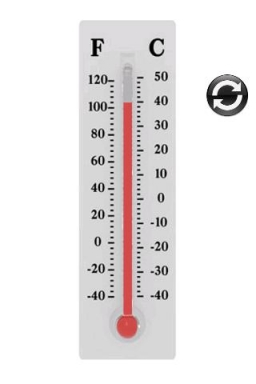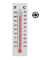# Difference of temperatures

The temperature on Saturday was –2°C. The temperature on Sunday was 8°C. Write down the difference between these two temperatures.

d =  10 °C

### Step-by-step explanation:Did you find an error or inaccuracy? Feel free to write us. Thank you!## Related math problems and questions:

• The lowestThe lowest temperature on Thursday was 20°C. The lowest temperature on Saturday was 12°C. What was the difference between the lowest temperatures?
• The temp rangeWhat is the range in temperatures if the high temperatures is 67 degrees Celsius and the low temperature is -25 degrees Celsius?
• Difference in temperaturesThe temperature in the number line show minus 15 degree celsius and 8 degree celsius. What is the difference between the temperatures?
• The temperature 11The temperature on Sunday was 4°C. The temperature dropped 8°C on Monday and twice as much on Tuesday. What is the temperature on Tuesday?
• Temperature rise and fallOn Friday, the temperature was 82°F. The temperature changed by –2°F on Saturday, and then it changed by 5°F on Sunday. What was the temperature on Sunday? How did the temperature change? Note we consider the mathematical problem formulated in this way to
• Temperature 2Sunday's high temperature was 3 degrees higher than Saturday's. On Monday, the temperature fell 5 degrees, then rose 7 degrees on Tuesday and 4 more on Wednesday. Then it fell 17 degrees to a record low of 31 on Thursday. What was the temperature on Satur
• Temperature 13Work out the temperature after each change. The temperature starts at six °C and falls by 13°C. The temperature starts at two °C and falls by eight °C
• The temperature 8The temperature on Monday was –6°C. On Tuesday, the temperature was 3 degrees lower. Write down the temperature on Tuesday.
• WeatherWeather. At 5:00 am, the temperature was 28°C, and at noontime, it rose by 9°C. By 4:00 pm, it dropped 3°C, and by nighttime, it was 4°C less. What is the room temperature this time?
• LaboratoryMang Elio went to his laboratory at 7:00am And recorded that the temperature was 11.07°C. At lunchtime, the temperature rose by 27.9°C but dropped by 8.05°C at 5:00 PM. What was the temperature at 5:00 PM?
• Now isNow is 5°C morning. What will the temperature be if i. It rises by 12°C ii. It falls by 15°C
• Temperature up and dowmAt 6 AM, the temperature is 19.4°C. Between 6 AM and noon, the temperature rises 3.8°C. Between noon and 6 PM, the temperature falls 2.5°C. What is the temperature at 6 PM?
• Temperature difference 2The temperature in London on new year’s day is -2 degree Celsius. The temperature in Moscow on the same day is -14 degree Celsius, what is the temperature difference between the two cities?
• Temperature differenceLibya's highest temperature was recorded at 58 degrees Celsius, and the lowest was recorded at -88 degrees Celsius. What is the temperature difference?
• Change in temperatureStarting temperature is 21°C, the highest temperature is 32°C. What is the change in temperature?
• At midmorningThe temperature at midmorning was 31°C. Early in the evening, the temperature dropped by 5°C. What was the resulting temperature?
• Temperature variationsToday's temperature was 80 degrees, and then the temperature dropped 10 degrees. Then it dropped 15 degrees again, then the next day, the temperature went up 2 degrees. What would the temperature be?